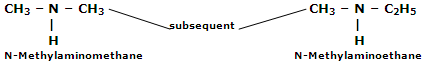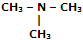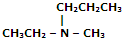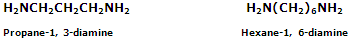What are aliphatic amines and its nomenclature?

In common system, the aliphatic amines are named by using prefix for alkyl group followed by the word amine.

In case of mixed amines, the name of alkyl groups are arranged in alphabetical order. This is followed by the word amine. However, for simple secondary or tertiary amines another prefix di or tri is added before the name of the alkyl group.

There is yet another system of naming amines according to which they are called aminoalkanes. In this system, the secondary or tertiary amines are named as N-alkylaminoalkanes. In this case, the smaller alkyl groups are taken as subsequent on nitrogen atom of primary amine. For example,In IUPAC system, the amines are considered to be amino derivatives of corresponding alkanes. Therefore, they are called alkanamines. These names are obtained by replacing e from the name of the parent alkane with suffix amine. The secondary and tertiary amines are, however, named as N-substituted derivatives of largest group of primary amines, i.e. N-Alkylalkanamines. The common and IUPAC names of some simple amines are given below in tabular form.

 Amine Common Name IUPAC Name 1° Amines CH3NH3 Methyl amine Methanamine CH3CH2NH2 Ethyl amine Ethanamine CH3CH2CH2NH2 n-Propyl amine Propan-1-amine (CH3)2CHNH2 Iso-Propyl amine Propan-2-amine 2° Amines CH3 - NH - CH3 Dimethyl amine N-Methyl-methanamine CH3 - NH - C2H5 Ethyl methyl amine N-Methyl-ethanamine 3° AminesTri-methyl amine N, N-Dimethyl-methanamineEthyl methyl amine N-Ethyl-N-methyl-propanamine

For compounds containing more than one amino group, a prefix di, tri, etc. is added before the suffix amine and the terminal e of the name of the hydrocarbon part is retained.#### Related Questions in Chemistry

• ##### Q :Molar concentration of hydrogen 20 g of

20 g of hydrogen is present in 5 litre of vessel. Determine he molar concentration of hydrogen: (a) 4  (b) 1 (c) 3 (d) 2 Choose the right answer from above.

• ##### Q :Liquid surfaces The surface between a

The surface between a liquid and a vapour distinguishes these fluids. The surface tension of liquids can be looked upon as that the property which draws a liquid together and forms a liquid vapour interface, therefore, distinguishing liquids from gases.<

• ##### Q :Mole fraction in vapours Choose the

Choose the right answer from following. If two substances A and B have P0A P0B= 1:2 and have mole fraction in solution 1 : 2 then mole fraction of A in vapours: (a) 0.33 (b) 0.25 (c) 0.52 (d) 0.2

• ##### Q :Problem on relative humidity Relative

Relative humidity is the ratio of the partial pressure of water in air to the partial pressure of water in air saturated with water at the same temperature, stated as a percentage: Relative  =

##### Q :Problem on decinormal Select the right

Select the right answer of the question. How much water is required to dilute 10 ml of 10 N hydrochloric acid to make it exactly decinormal (0.1 N): (a) 990 ml (b) 1000 ml (c) 1010 ml (d) 100 ml

• ##### Q :Liquid Vapour Free Energies The free

The free energy of a component of a liquid solution is equal to its free energy in the equilibrium vapour.Partial molal free energies let us deal with the free energy of the components of a solution. We use these free energies, or simpler concentration ter

• ##### Q :Raoults law Give me answer of this

Give me answer of this question. Provide solution of this question. Which one of the following is the expression of Raoult's law: (a) P-P1/P = n/n+N (b) P1-P/P = N/ N+n (c)P-P2/P1= N/ N-n (d) P1-P/P2= N-n/N

• ##### Q :Describe First Order Rate Equation The

The integrated forms of the first order rate equations are conveniently used to compare concentration time results with this rate equation. Rate equations show the dependence of the rate of the reaction on concentration can be integrated to give expressions fo

• ##### Q :Cations Chromium(III) hydroxide is

Chromium(III) hydroxide is highly insoluble in distilled water but dissolves readily in either acidic or basic solution. Briefly explain why the compound can dissolve in acidic or in basic but not in neutral solution. Write appropriate equations to support your answer